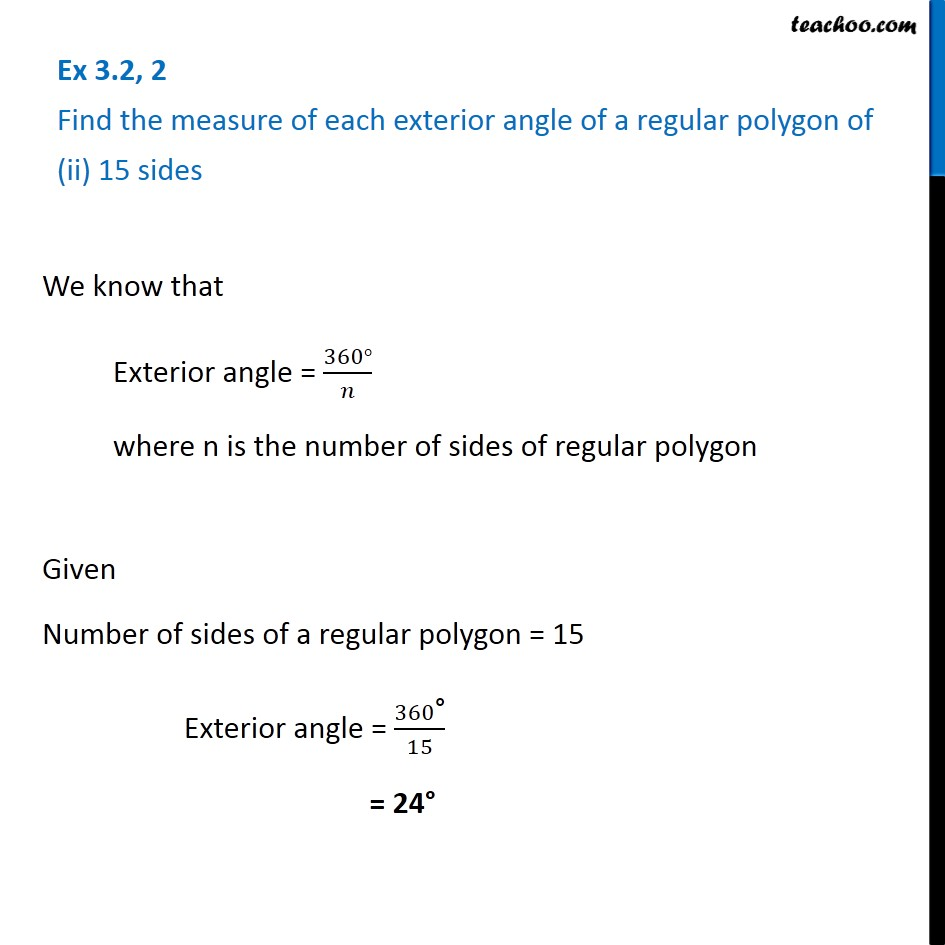Ex 3.2

Chapter 3 Class 8 Understanding Quadrilaterals
Serial order wiseLearn in your speed, with individual attention - Teachoo Maths 1-on-1 Class

### Transcript

Ex 3.2, 2 Find the measure of each exterior angle of a regular polygon of (ii) 15 sidesWe know that Exterior angle = (360°)/𝑛 where n is the number of sides of regular polygon Given Number of sides of a regular polygon = 15 Exterior angle = 360"°" /15 = 24°